Next: References Up: Steady flows of incompressible Previous: Aplications of Bernoulli

## Drag and D'Alembert's "paradox"

Let's now consider one particularly simple nonviscous flow, the irrotational flow of a fluid around a cylinder. This problem will demonstrate how some fluid mechanics problems are solved, and brings out an important paradox in the theory of nonviscous flow.

We consider a cylinder of radius R, with an imposed flow of velocity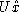far from the cylinder--see Fig. 2.7.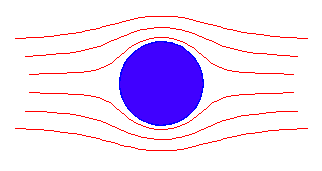Figure 2.7: Flow past a cylinder

.
We know that the fluid cannot penetrate the surface of the cylinder, so that the normal component of the velocity at the surface of the cylinder must be zero. In radial coordinates this is expressed as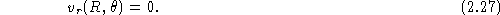What is the equation for? Since the flow is irrotational,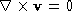, and from vector calculus we know that this implies thatmay be written as the gradient of some scalar function: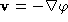. If the fluid is also incompressible, then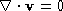, so that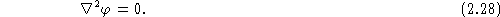The potential function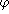is a solution of Laplace's equation, which should be familiar to those of you who have studied advanced electrostatics. In radial coordinates Laplace's equation is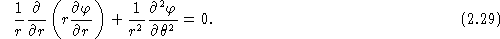In terms ofthe boundary condition at infinity is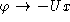; in radial coordinates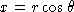, so this becomesAt the surface of the cylinder we have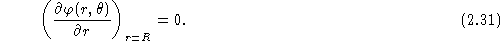The strategy is that we solve Laplace's equation for, and then calculate the velocity field from the potential.1 To do this we first need to realize that in order to satisfy the boundary condition at infinity,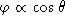; therefore, we set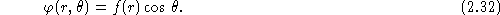Substituting this into Laplace's equation, Eq. (2.29), we obtain a differential equation for f: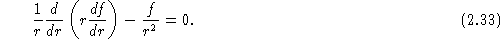To solve this equation, we'll guess the solution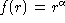, and see if we can find an appropriate value of. Substituting, we find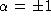, so that our solution isUsing the boundary condition at infinity, we see that A=-U; applying the boundary condition at the surface of the cylinder, we find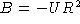, so our final solution isThe components of the velocity are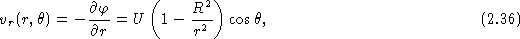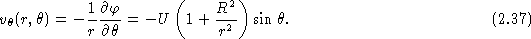The streamlines in this case are symmetric on the upstream and downstream sides of the cylinder.

How does the pressure vary around the cylinder? First, the square of the velocity on the surface of the cylinder is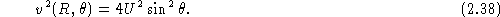We can now use Bernoulli's equation; since the flow is irrotational, the constant is the same everywhere in the fluid. In particular, at infinity the constant is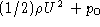, where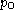is the ambient pressure (which we can choose to be zero without any loss of generality). The pressure on the surface of the cylinder is thenFrom the pressure we can calculate the net force acting on the cylinder. First, recall that the pressure at the surface of the cylinder is the force per unit area acting on the cylinder; therefore the force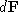acting upon an element of the cylinder of surface area dS is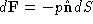, where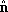is a unit vector normal to the surface, and the minus sign indicates that this force acts inward. Next, we integrate this over the entire surface of the cylinder to find the net force: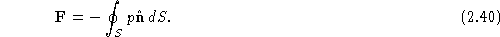For the cylinder,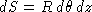, with z along the axis of the cylinder. The unit vector depends upon the angleas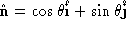. Then the x and y components of the force are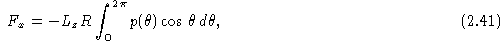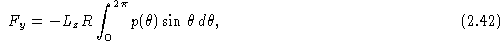where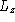is the length of the cylinder in the z-direction. If we now substitute our expression for the pressure distribution on the surface of the cylinder, Eq. 2.39, into our expressions for the drag and the lift, Eqs. 2.41 and 2.42, and perform theintegration, we find that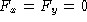! Although we have performed an explicit calculation for a cylinder, the result is more general: irrotational flow of a nonviscous fluid about an object produces no drag on the object. This peculiar result is known as d'Alembert's paradox.

Physically, the fact that the drag is zero is due to the symmetry of the pressure field about the cylinder--the fluid is pushing as hard on the upstream side of the cylinder as on the downstream side, so there are no unbalanced forces which would lead to drag. Whether or not this is paradoxical is a matter of opinion. If there were a drag on the cylinder, how would the energy be dissipated in a nonviscous fluid? To really understand drag, we need to go beyond the nonviscous approximation and treat real fluids.

`Footnote`1 In electrostatics j would be the electrostatic potential, and the electric field would be given by E = - Ñj.Next: References Up: Steady flows of incompressible Previous: Applications of Bernoulli

Notes by A. Dorsey, revised by V. Celli, U. of Virginia
Thu Oct 12 01:02:02 EDT 1997